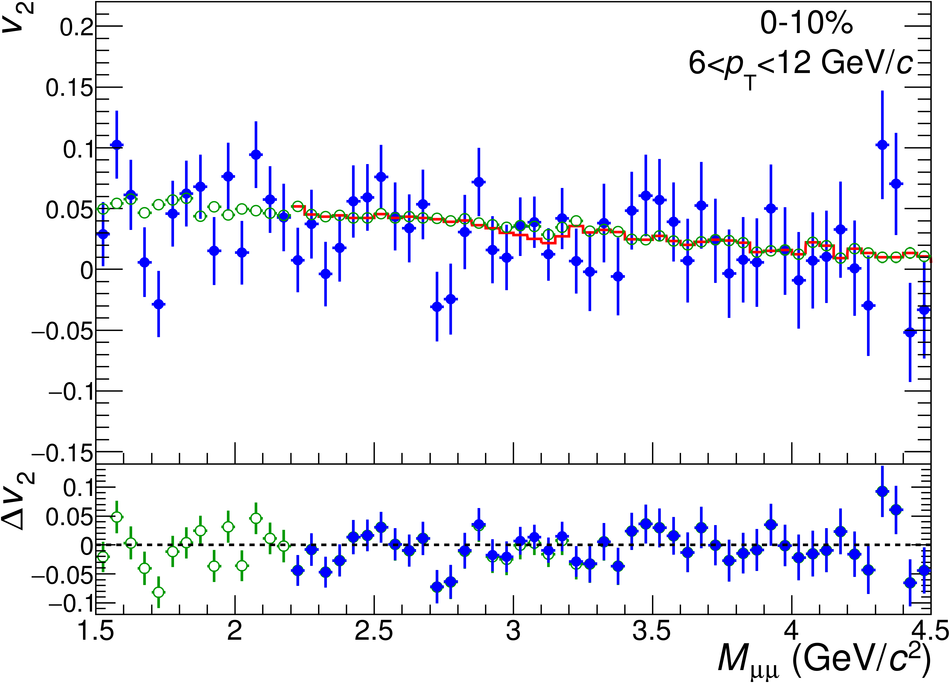# Figure 2

 The $v_2(M_{\mu\mu})$ distribution in low (top panels), intermediate (middle panels) and high (bottom panels) $p_{\rm T}$ intervals for central (left panels) and semi-central (right panels) collisions. The data are fitted with the function from Eq.~\ref{eq:vn_sb}, where the background coefficient $v_2^{\rm B}(M_{\mu\mu})$ is fixed using the event-mixing procedure. The background coefficient $v_2^{\rm B}(M_{\mu\mu})$ alone down to 1.5 GeV/$c^2$ is also presented. Only statistical uncertainties are shown.